7 Twins and TripletsV'ir Tbg n FrpergThis document as PDF9 Adieu

# 8 The Hill Cipher

Rather than working with such large numbers, the Hill cipher works on groups of letters in a somewhat different manner. The Hill cipher works by viewing a group of letters as a vector, and encryption is done by matrix multiplication. However, we will avoid reference to linear algebra in our explanation.

The Hill cipher was first described by Lester Hill in a paper published in 1931. While this cipher can work on blocks of letters of any length, we'll describe it as working on pairs of letters, or digraphs.

First, our key consists of four numbers which we call a, b, c, and d. These numbers must be chosen so that the quantity ad-bc is relatively prime to the length of the alphabet (26 in our case, so ad-bc cannot be even or a multiple of 13).

To encrypt a pair of letters, we look up their numeric equivalents as usual. Suppose these numbers are x and y. Then the corresponding letters in the ciphertext are given byAs an example, let's encipher the phrase do it now using a Hill Cipher with the key a=2, b=3, c=5, and d=6. Since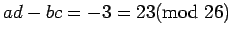, this is a valid key. To encode, we break our text up into pairs, and since there are an odd number of letters, we add x to the end.

 plaintext do it no wx (3, 14) (8, 19) (13,14) (22,23) (ax+by, (6+42=22 (16+57=21, (26+42=16, (44+69=9, cx+dy) 15+84=21) 40+114=24) 65+84=19) 110+138=14 ciphertext WV VY QT JO

To decipher, we apply a different Hill cipher to the ciphertext. If the pairs of letters have numeric equivalencies X and Y, then the plaintext is given byTo find A, B, C, and D, we first find the multiplicative inverse of ad-bc and denote it by J. Then12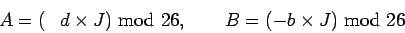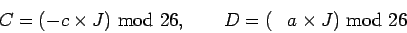For example, if we encipher with a=2, b=3, c=5, and d=6 as above, then J=17, and the deciphering transformation has A=24, B=1, C=19, and D=8.

The real strength of the Hill cipher is that it can be adapted to work on large blocks of text without having to use huge numbers as we did in the previous section. For example, to work on blocks of 3 letters at a time, we choose 9 numbers a,b,c, d,e,f, g, h, k, and then the code of the ciphertext corresponding to the triple (x,y,z) is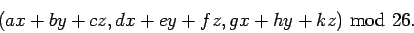Extending this to larger blocks of letters works analogously.

In attempting to crack a Hill cipher, the usefulness of frequency analysis becomes vanishingly small as the size of the blocks of letters increases. A Hill cipher isn't hard to implement on groups of 6 or more letters. A major disadvantage, however, is that it is quite susceptible to what is called a known plaintext attack. If part of the plaintext is known to the attacker, then the deciphering transformation can be deduced merely by solving some linear equations. Some help can be gained by applying a Vigenère cipher after encrypting with a Hill cipher,13although there are much better ways to improve the security.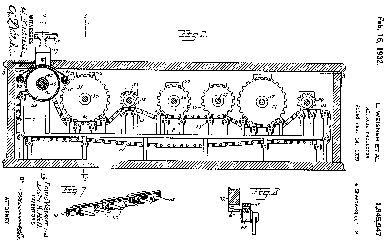It is possible to build a machine that does all of the arithmetic corresponding to the Hill cipher by means of gears and pulleys. Lester Hill and Louis Weisner were issued a patent for a Message Protector'' which mechanically implemented a Hill cipher acting on blocks of six letters at a time. The major gears in the machine correspond to a number circle'' as discussed on page; multiplication is done by advancing the gear by a fixed number of teeth several times (that is, repeated addition). The figure is taken from the patent application (adapted from [Cdb]).

#### Footnotes

... Then12
This formula comes from linear algebra. It is just the inverse of the matrix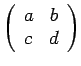.
... cipher,13
If the length of the key for the Vigenère cipher is the same as the length of the letter-blocks, this yields an affine cipher AX+B, where A is an n x n matrix, X is our vector of n plaintext letters, and B is the n-vector corresponding to the Vigenère key.7 Twins and TripletsV'ir Tbg n FrpergThis document as PDF9 Adieu
Scott Sutherland
2005-10-26